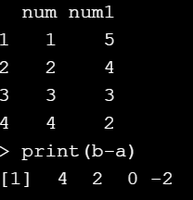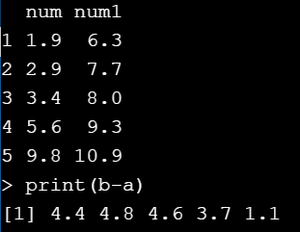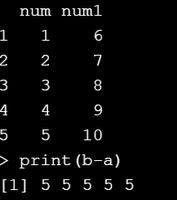# Calculate difference between columns of R DataFrame

• Last Updated : 26 Jan, 2022

Generally, the difference between two columns can be calculated from a dataframe that contains some numeric data. In this article, we will discuss how the difference between columns can be calculated in the R programming language.

Approach

• Create a dataframe and the columns should be of numeric or integer data type so that we can find the difference between them.
• Extract required data from columns using the \$ operator into separate variables. For example, we have two columns then extract individual columns into separate variables.
• Then perform the minus operation for the difference between those columns.
• Finally, print the result.

Example 1 :

## R

 `# creating a dataframe``df=``data.frame``(num=``c``(1,2,3,4),num1=``c``(5,4,3,2)) ` `# Extracting column 1``a=df\$num` `# Extracting column 2``b=df\$num1` `# printing dataframe``print``(df)` `# printing difference among``# two columns``print``(b-a)`

Output :Example 2 :

## R

 `# creating a dataframe with some``# numeric data``df=``data.frame``(num=``c``(1.9,2.9,3.4,5.6,9.8),``              ``num1=``c``(6.3,7.7,8.0,9.3,10.9))``print``(df)` `# extracting column 1 into a``# variable called a``a=df\$num` `# extracting column 2 into a``# variable called b``b=df\$num1` `# printing the difference between``# two columns``print``(b-a)`

Output :Example 3 :

## R

 `# creating a dataframe with``# some numeric data``df=``data.frame``(num=``c``(1,2,3,4,5),``              ``num1=``c``(6,7,8,9,10))` `# extracting column 1 into a``# variable called a``a=df\$num` `# extracting column 2 into a``# variable called b``b=df\$num1` `# printing the dataframe``print``(df)` `# printing the difference``# between two columns``print``(b-a)`

Output :My Personal Notes arrow_drop_up